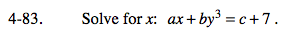### Home > A2C > Chapter 4 > Lesson 4.2.1 > Problem4-83

4-83.Subtract by3 from both sides of the equation.

ax = c + 7 − by3

Now finish solving for x by dividing both sides by a.

$\textit{x}=\frac{\textit{c}+7-\textit{by}^{3}}{\textit{a}}$